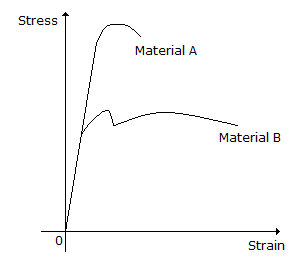# Civil Engineering - GATE Exam Questions

### Exercise :: GATE Exam Questions - Section 6

11.

The stress-strain diagram for two materials A and B is shown below.The following statements are made based on this diagram :

1. Mateiral A is more brittle than material B.
2. The ultimate strength of material B is more than that of A.

With reference to the above statements, which of the following applies ?

 A. Both the statements are false. B. Both the statements are true. C. I is true but II is false. D. I is false but II is true.

Explanation:

No answer description available for this question. Let us discuss.

12.

Base course is used in rigid pavements for

 A. prevention of subgrade settlement B. prevention of slab cracking C. prevention of pumping D. prevention of thermal expansion

Explanation:

No answer description available for this question. Let us discuss.

13.

A reinforced concrete structure has to be constructed along a sea coast. The minimum grade of concrete to be used as per IS : 456-2000

 A. M 15 B. M 20 C. M 25 D. M 30

Explanation:

No answer description available for this question. Let us discuss.

14.

The relationship between the length (l) and radius (r) of an ideal transition curve is given by

 A. l ∝ r B. l ∝ r2 C. l ∝ 1/r D. l ∝ 1/r2

Explanation:

No answer description available for this question. Let us discuss.

15.

The width of expansion joint gap is 2.5 cm in a cement concrete pavement. The spacing between expansion joints for a maximum rise in temperature of 25°C is, (assuming coefficient of thermal expansion of concrete as 10 x 10-6 per degree C),

 A. 5 m B. 50 m C. 100 m D. 25 M